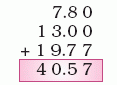Name: ___________________Date:___________________

 Email us to get an instant 20% discount on highly effective K-12 Math & English kwizNET Programs!

 Write the decimals one below the other. Note that the point must be one below the other and the numbers one below the other. Add the numbers. Put the decimal point for the sum. Example: Add 7.88, 13, 19.77Here the decimals 7.88 and 19.77 have two decimal places. So 13 is written as 13.00 . Add the decimals as shown. Answer: 40.57 Directions: Add the following. Also write at least 10 examples of your own.
 Question 1: 875.4 + 936.3

 Question 2: 1.699 + 2.667

 Question 3: 95.84 + 91.23

 Question 4: 5.945 + 1.015

 Question 5: 60.52 + 90.73

Question 6: This question is available to subscribers only!

Question 7: This question is available to subscribers only!

Question 8: This question is available to subscribers only!

Question 9: This question is available to subscribers only!

Question 10: This question is available to subscribers only!